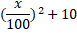# Quiz Discussion

In a family, the average age of a father and a mother is 35 years. The average age of the father, mother and their only son is 27 years. What is the age of the son?

Course Name: Quantitative Aptitude

• 1] 10 years
• 2] 10.5 years
• 3] 11 years
• 4] 12 years
##### Solution
No Solution Present Yet

#### Top 5 Similar Quiz - Based On AI&ML

Quiz Recommendation System API Link - https://fresherbell-quiz-api.herokuapp.com/fresherbell_quiz_api

# Quiz
1
Discuss

Ajay working in a Cellular company as a salesman. His monthly salary is Rs. 200. But he gets bonus as per given rule. If he sells sim cards of Rs. X then his bonus will be. In the first quarter of the year his average sale was Rs. 3000 per month. In the next 5 five month his average sale was Rs. 5000 per month and for next four month his average sale was Rs. 8000 per month. What is the average earning per month for the whole year?

• 1] Rs. 3350
• 2] Rs. 3610
• 3] Rs. 3750
• 4] Rs. 3560
• 5] None of these
##### Solution
2
Discuss

Find the average of the first 40 natural numbers.

• 1]

21

• 2]

20.5

• 3]

21.5

• 4]

None Of These

##### Solution
3
Discuss

In 2011, the arithmetic mean of the annual incomes of Ramesh and Suresh was Rs. 3800. The arithmetic mean of the annual incomes of Suresh and Pratap was Rs. 4800, and the arithmetic mean of the annual incomes of Pratap and Ramesh was Rs. 5800. What is the arithmetic mean of the incomes of the three?

• 1] Rs. 4000
• 2] Rs. 4200
• 3] Rs. 4400
• 4] Rs. 4800
##### Solution
4
Discuss

The average of two numbers is XY. If one number is X, the other is-

• 1]

Y/2

• 2]

Y

• 3]

2XY - X

• 4]

X (Y - 1)

##### Solution
5
Discuss

Five years ago, the average age of P and Q was 25. The average age of P, Q and R today is 25. Age of R after 5 years will be :

• 1] 15 years
• 2] 20 years
• 3] 40 years
• 4] 35 years
##### Solution
6
Discuss

A student finds the average of 10, 2 digits numbers. If the digits of one of the numbers interchanged, the average increases by 3.6. The difference between the digits of the 2 digits number is :

• 1] 4
• 2] 3
• 3] 2
• 4] 5
##### Solution
7
Discuss

The average of 11 results is 60. If the average of first six results is 58 and that of last six is 63, find the 6th result-

• 1] 66
• 2] 55
• 3] 64
• 4] 68
##### Solution
8
Discuss

If the arithmetic mean of 0, 5, 4, 3 is a, that of -1, 0, 1, 5, 4, 3 is b and that of 5, 4, 3 is c, then the relation between a, b, and c is

• 1] a = b = c
• 2] a : b : c = 3 : 2 : 4
• 3] 4a = 5b = c
• 4] a + b + c = 12
##### Solution
9
Discuss

The average age of a family with 5 members is 28. If one of the 5 members of age 20 is excluded the average age of the family becomes -

• 1] 25
• 2] 20
• 3] 30
• 4] 24
##### Solution
10
Discuss

Find the average of all the numbers between 6 and 34 which are divisible by 5 -

• 1] 18
• 2] 20
• 3] 24
• 4] 30
# Quiz